# Constructing Some Classes of Specialized Numerical O.D.E. Integrators by Means of Computer Algebra Systems

Nikolay O. Kirsanov, Nikolay N. Vassiliev
Institute for Theoretical Astronomy of RAS
St. Petersburg, Russia

Extended Abstract We consider the methods for numerical integration of O.D.E. as the methods for approximation of shift map along trajectories of these O.D.E. From this point of view we can study such approximation maps by numerical and analytical means. Highly developed computer algebra systems allow us to construct the one-step maps for the defined numerical methods and for the defined systems of O.D.E. purely in analytical mode. Taking into account all specific features of the given O.D.E. we can in many cases use additional possibilities. A simple approach to one-step high-order method construction lies in the Taylor's development of the solution into a power series. We considered ([3, 4]) alike method [1, 2] for solving the system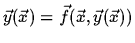: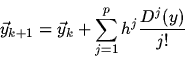(1)

where y is the solution (we assume that f is analytical), h is a step, D is a differential operator along the trajectories of the system. When p = 1 this method is simply the Euler's method and one may see easily that such a method has the order p, i. e. it has the global method error o(hp). Note that the higher derivatives of the solution one may determine from the system itself. The simplicity of constructing the method allowed us to build a Reduce program with a rather comfortable interface, giving opportunities to straight-forward input of the equation and initial data within the frames of an interactive session. The behavior of the integrator at respect of its parameters were easily investigated online and compared with the 'hand-made' Fortran integrators of  (ours won). Usually the complexity of a method (and, in particular, of the method (1)) grows when the method order grows. For our case it is apparent from the formula (1), because the method is a polynomial with the power p with respect of h and it takes into account all the derivatives of the right-hand parts up to the power p. But when we put into (1) analytical right-hand parts and the numerical value of h, we can see that there exists a class of O.D.E. systems such that the one-step map for a method of the p-th order is not more complex than this for the first order. For example, all the linear systems of O.D.E. belong to this class - it is known that precise solutions of these satisfy the formula: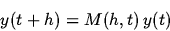(2)

where M is some matrix, depending on the integration step. If we use the formula (2) for the linear equation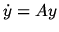, we'll obtain a sequence of difference formulae like: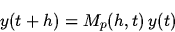(3)

where p is the power of the method. When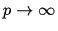, we obtain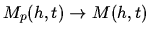. Using the explicit formula for one step map for a Taylor-like integrator for solving non-linear Henon-Heiles equations  one could have the simple formula for total degree of the components of this map: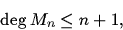(4)

which means that for the Taylor-like integrator of order n the total degree of the components of the method's one-step map is equal or less than n + 1. This is obviously better than in the case of non-specialized methods. The specializing methods obviously have not to be linked with Taylor-like methods, but it seems useful to keep the 'recursiveness' of constructing the method. This can greatly help us to construct integrators for specialized classes of O.D.E., e. g. this approach can be used to construct symplectic integrators for Hamiltonian systems. Our formulae thus can be used for an estimation of complexity of evaluation of the one-step map for a given Hamiltonian and consequently for the estimation of the effectiveness of the procedure of the numerical integration. References
1.
Ortega J. M., Poole W. G., Jr. An Introduction to Numerical Methods for Differential Equations. - Pitman Publishing Inc., 1981.
2.
Roberts C. E., Jr. Ordinary differential Equations. A Computational Approach. - Englewood Cliff's, N.Y.: Prentice-Hall Inc., 1979.
3.
Vassiliev N. N., Kirsanov N. O. Producing the High Power One-Step Methods in Analytical Form to Integrate Asteroid Motions // Asteroid Hassard-95: Abstracts of the Conference. (S.Petersburg,, 23-25 May 1995). ITA RAS publishing, edited by A.G.Sokolsky, 1, (1995), pp. 20 - 22.
4.
Vassiliev N. N., Kirsanov N. O. Producing the High Power One-Step Methods in Analytical Form to Integrate Henon-Heiles Equations // Structure and Evolution of Stellar Systems: Proc. of the Conference. Petrozavodsk, August 13-17, 1995. - St. Petersburg, (1997), pp. 4l-44.

IMACS ACA'98 Electronic Proceedings